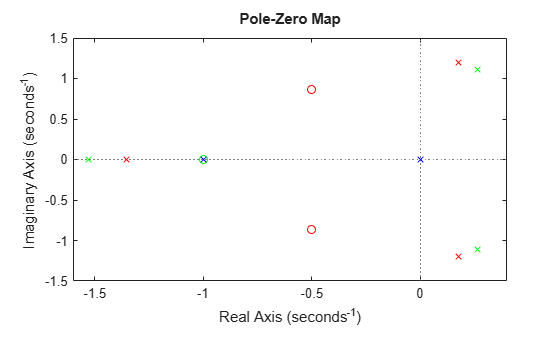# pzplot

Pole-zero plot of dynamic system model with additional plot customization options

## Syntax

``h = pzplot(sys)``
``h = pzplot(sys1,sys2,...,sysN)``
``h = pzplot(sys1,LineSpec1,...,sysN,LineSpecN)``
``h = pzplot(ax,...)``
``h = pzplot(...,plotoptions)``

## Description

`pzplot` lets you plot pole-zero maps with a broader range of plot customization options than `pzmap`. You can use `pzplot` to obtain the plot handle and use it to customize the plot, such as modify the axes labels, limits and units. You can also use `pzplot` to draw a pole-zero plot on an existing set of axes represented by an axes handle. To customize an existing plot using the plot handle:

1. Obtain the plot handle

2. Use `getoptions` to obtain the option set

3. Update the plot using `setoptions` to modify the required options

For more information, see Customizing Response Plots from the Command Line (Control System Toolbox). To create pole-zero maps with default options or to extract pole-zero data, use `pzmap`.

example

````h = pzplot(sys)` plots the poles and transmission zeros of the dynamic system model `sys` and returns the plot handle `h` to the plot. `x` and `o` indicates poles and zeros respectively.```

example

````h = pzplot(sys1,sys2,...,sysN)` displays the poles and transmission zeros of multiple models on a single plot. You can specify distinct colors for each model individually.```

example

````h = pzplot(sys1,LineSpec1,...,sysN,LineSpecN)` sets the line style, marker type, and color for the plot of each system. All systems must have the same number of inputs and outputs to use this syntax.```
````h = pzplot(ax,...)` plots into the axes specified by `ax` instead of the current axis `gca`.```

example

````h = pzplot(...,plotoptions)` plots the poles and transmission zeros with the options specified in `plotoptions`. For more information on the ways to change properties of your plots, see Ways to Customize Plots (Control System Toolbox).```

## Examples

collapse all

Plot the poles and zeros of the continuous-time system represented by the following transfer function:

`$sys\left(s\right)=\frac{2{s}^{2}+5s+1}{{s}^{2}+3s+5}.$`

```sys = tf([2 5 1],[1 3 5]); h = pzplot(sys); grid on```Turning on the grid displays lines of constant damping ratio (zeta) and lines of constant natural frequency (wn). This system has two real zeros, marked by o on the plot. The system also has a pair of complex poles, marked by x.

Change the color of the plot title. To do so, use the plot handle, `h`.

```p = getoptions(h); p.Title.Color = [1,0,0]; setoptions(h,p);```For this example, load a 3-by-1 array of transfer function models.

```load('tfArrayMargin.mat','sys'); size(sys)```
```3x1 array of transfer functions. Each model has 1 outputs and 1 inputs. ```

Plot the poles and zeros of the model array. Define the colors for each model. For this example, use red for the first model, green for the second and blue for the third model in the array.

`pzplot(sys(:,:,1),'r',sys(:,:,2),'g',sys(:,:,3),'b');`Plot the poles and zeros of the continuous-time system represented by the following transfer function with a custom option set:

`$sys\left(s\right)=\frac{2{s}^{2}+5s+1}{{s}^{2}+3s+5}.$`

Create the custom option set using `pzoptions`.

`plotoptions = pzoptions;`

For this example, specify the grid to be visible.

`plotoptions.Grid = 'on';`

Use the specified options to create a pole-zero map of the transfer function.

`h = pzplot(tf([2 5 1],[1 3 5]),plotoptions);`Turning on the grid displays lines of constant damping ratio (zeta) and lines of constant natural frequency (wn). This system has two real zeros, marked by `o` on the plot. The system also has a pair of complex poles, marked by `x`.

## Input Arguments

collapse all

Dynamic system, specified as a SISO or MIMO dynamic system model, or an array of SISO or MIMO dynamic system models. Dynamic systems that you can use include continuous-time or discrete-time numeric LTI models such as `tf` (Control System Toolbox), `zpk` (Control System Toolbox), or `ss` (Control System Toolbox) models.

If `sys` is a generalized state-space model `genss` or an uncertain state-space model `uss`, `pzplot` returns the poles and transmission zeros of the current or nominal value of `sys`. If `sys` is an array of models, `pzplot` plots the poles and zeros of each model in the array on the same diagram.

Line style, marker, and color, specified as a character vector or string containing symbols. The symbols can appear in any order. You do not need to specify all three characteristics (line style, marker, and color). For example, if you omit the line style and specify the marker, then the plot shows only the marker and no line.

Example: `'--or'` is a red dashed line with circle markers

Line StyleDescription
`-`Solid line
`--`Dashed line
`:`Dotted line
`-.`Dash-dot line
MarkerDescription
`'o'`Circle
`'+'`Plus sign
`'*'`Asterisk
`'.'`Point
`'x'`Cross
`'_'`Horizontal line
`'|'`Vertical line
`'s'`Square
`'d'`Diamond
`'^'`Upward-pointing triangle
`'v'`Downward-pointing triangle
`'>'`Right-pointing triangle
`'<'`Left-pointing triangle
`'p'`Pentagram
`'h'`Hexagram
ColorDescription

`y`

yellow

`m`

magenta

`c`

cyan

`r`

red

`g`

green

`b`

blue

`w`

white

`k`

black

Axes handle, specified as an axes object. If you do not specify the axes object, then `pzplot` uses the current axes `gca` to plot the poles and zeros of the system.

Pole-zero plot options, specified as an options object. See `pzoptions` for a list of available plot options.

## Output Arguments

collapse all

Pole-zero plot options handle, returned as a scalar. Use `h` to query and modify properties of your pole-zero plot. You can use this handle to customize the plot with the `getoptions` and `setoptions` commands.

## Version History

Introduced before R2006a